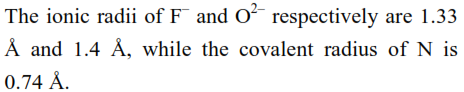`
Question:The correct statement for the ionic radius of $\mathrm{N}^{3-}$ from the following is :

1. It is smaller than $\mathrm{F}^{-}$and $\mathrm{N}$

2. It is bigger than $\mathrm{O}^{2-}$ and $\mathrm{F}$

3. It is bigger than $\mathrm{F}^{-}$and $\mathrm{N}$, but smaller than of $\mathrm{O}^{2-}$

4. It is smaller than $\mathrm{O}^{2-}$ and $\mathrm{F}^{-}$, but bigger than of $\mathrm{N}$

Correct Option: , 2

Solution:

$\mathrm{F}^{-}, \mathrm{O}^{2-}$ and $\mathrm{N}^{3-}$ all are isoelectronic species in which $\mathrm{N}^{3-}$ have least number of protons due to which it's size increases as least nuclear attraction is experienced by the outer shell electrons.

Size order $\quad \mathrm{N}^{3-}>\mathrm{O}^{2-}>\mathrm{F}^{-}$